Home Curatorial Essays Phil Huneke

## Phil Huneke

-- in "Drawing on Sculpture" Bertha Urdang Gallery, 1979.p. 15.. Catalogue.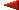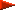"Smooth Tesselations of a Sphere and Other Surfaces"

Derivation

The 2-sphere is smoothly tesselated by two copies of a circular disc D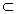2; that is, isometrically identifying the two boundary circles of D makes a 2-sphere, which can be embedded inm (m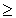3) with a smooth arc-length prserving embedding. Two copies of the disc D1 = [Fig. 2] smoothly tesselate the real projective plane by: [Fig. 3], with opposite points of the circle identified. Also, four copies of this same Yin Yang shape D1 smoothly tesselate a 2-sphere.

Mathematical definitions for a smooth tesselation of a surface by copies of a disc Dn2 (which has smooth boundary except for n peaked cusps) were inspired by inflatable models of smooth tesselations of the topological 2-sphere developed by Betty Collings. Each sculpture in her Con(jug)ation series uses two copies of a smooth disc D0 = [Fig. 4], while those in Anolatabulata each use four copies of D1 above. Betty Collings, in these two series, descovered all types of smooth tesselations of the 2-sphere according to the smooth tesselations of the 2-sphere.

Theorem.  If Dn2 is a disc with n peaked cusps which smoothly tesselates a surface M with p copies of Dn, then the Euler Characteristic of M is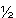p(2-n).

Hence the parameters n and p give the topological type of the surface of any such real world sculpture.

Corollary. The surface of a sculpture of a smooth tesselation is homeomorphic to a 2-sphere (equivalently, has Euler Characteristic 2) if and only if it is formed from either two dopies of D0 or four copies of D1.

Schematization

Betty Collings indicated all similar sculptures by using a circular scheme to display her Con(jug)ate series of sculptures. Any sculpture in this sereies can be continuously deformed into another so that each intermediate stage of the deformation gives an example of a smooth tesselation by two copies of D0. Mathematically, the set X0,2, of all smooth tesselations formed with two copies of D0 canonically corresponds to the set of all isometries of a circle, or equivalently, to the topological groupd of all real orthogonal 2x2 matrices. Hence X0,2 is two disjoint circles, each tessellation representing a position on a circle. The Con(jug)ation sculptures of Collings are thus naturally arranged on X0,2.

In general, the set Xn,p of all smooth tesselations of a surface M with p copies of a fixed disc Dn2 can be organized by a topology which is induced by continuous deformations of the tesselation. More mathematically precise definitions involve defining a topology on the set of equivalence relations on a topological space Y induced by the topology of Y.

As the parameters n and p increase, so do the degrees of freedom for the continuous deformations and hence also the complexity of the schema Xn,p. However, the spaces Xn,p seem to have geometrical descriptions as CW-complexes.

The component of X1,4 cataloguing all smooth tesselations of the 2-sphere with four copies of D1, all with the same orientation, has a two point compactification wihich is homeomorphic to Zeeman's Dunce cap (a contractable but not collapsable 2-complex) with a disc attached at its circular 1-skeleton. Twelve of Betty Collings' sculptures in the Anolatabulata series are represented as positions in this space, the others in this series sbelong in other analogous components of X1,4.

Phil Huneke. Professor of Mathematics, Ohio State University.

Click on images to enlarge.

 Home Curatorial Essays Phil Huneke